Courses

# Test: Fractions - 3

## 20 Questions MCQ Test Mathematics (Maths) Class 6 | Test: Fractions - 3

Description
This mock test of Test: Fractions - 3 for Class 6 helps you for every Class 6 entrance exam. This contains 20 Multiple Choice Questions for Class 6 Test: Fractions - 3 (mcq) to study with solutions a complete question bank. The solved questions answers in this Test: Fractions - 3 quiz give you a good mix of easy questions and tough questions. Class 6 students definitely take this Test: Fractions - 3 exercise for a better result in the exam. You can find other Test: Fractions - 3 extra questions, long questions & short questions for Class 6 on EduRev as well by searching above.
QUESTION: 1

### Which option gives an equivalent fraction of 13/25

Solution: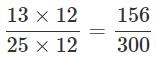QUESTION: 2

Solution:
QUESTION: 3

### What is the simplified form of the product 12/24 and 36/72

Solution:

12/24 x 36/72 = 1/2 x 1/2 = 1/4

QUESTION: 4

Give an example for a proper fraction.

Solution:

In a proper fraction, the numerator is less than the denominator.

QUESTION: 5

What is the fractional form of five eighteenths?

Solution:

The fractional form of five eighteenths

= 5/18

QUESTION: 6

Which fraction represents the shaded part of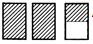?

Solution:

The shaded part represents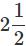QUESTION: 7

What are the fractions with the same denominator called?

Solution:

Like fractions have same denominator.

QUESTION: 8

Raju scored 9 marks in maths test. If the maximum marks of the test is 25, how is Raju's score represented as a fraction?

Solution:

Maximum marks = 25 Marks scored by Raju = 9

∴ Raju's score represented as a fraction

= 9/25

QUESTION: 9

Which of the following fractions is the largest?

Solution:

All fractions have the same numerator. So, the fraction having the smallest denominator is the largest.

∴ 29/23 is the required largest fraction.

QUESTION: 10

What is the value of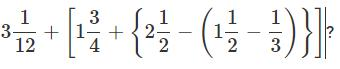Solution: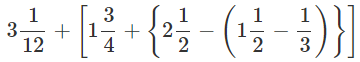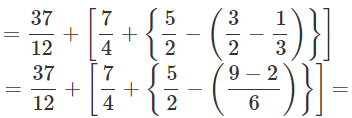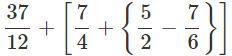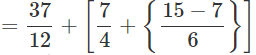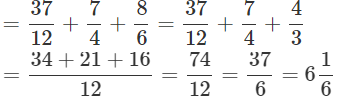QUESTION: 11

What should be added to 11/17 to make it 15/17?

Solution:

15/17 - 11/17 = 15 - 11/17 = 4/17

QUESTION: 12

By how much is 19/20 greater than 2/20?

Solution:

19/20 - 2/20  = 19 - 2/20 = 17/20

QUESTION: 13

What do you call fractions with different denominators?

Solution:

Unlike fractions have different denominators.

QUESTION: 14

What type of fraction is the sum 3/4 + 2/4?

Solution:

3/4 + 2/4 = 3+2/4 = 5/4 is an improper fraction. Since, the numerator is greater than the denominator.

QUESTION: 15

Which of these makes a whole?

Solution:
QUESTION: 16

In which of the following does the shaded part represent one fourth of its whole?

Solution:
QUESTION: 17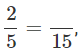What number should replace thein the given equation?

Solution:
QUESTION: 18

What are the fractions which have one as the numerator called?

Solution:
QUESTION: 19

How is the fractional number for "3 out of 7 of the fruits are apples" written?

Solution:
QUESTION: 20

Two fractions are equivalent if their cross multiplications are

Solution: